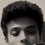# Non - conservation of linear momentum

Consider a projectile launched with a certain velocity.During it's time of flight, let it disintegrate into two masses.. Now, everyone of us knows that the centre of mass of the two masses continues to traverse the good old parabolic path(neglecting air resistance).

But, when we say that the momentum of the two body system remains conserved, we would indirectly mean that there is no net external force on the system. But we know that the c.o.m continues to accelerate, and also, we do know it's value(g). So, this would imply that the linear momentum of the system is not conserved during the disintegration, since however small be the time interval of the disintegration, the centre of mass keeps on accelerating. Some people told me that the gravitational force is non-impulsive during the disintegration, because the disintegration takes place in a very short interval of time, in which one has to consider only the internal forces(and thus leading to conservation of momentum of the system)

But, if you consider a situation where the the two parts are initially connected by a spring , and held rigid by another internal force until the arrival of the time of disintegration, when the spring is allowed to elongate pushing the blocks outward, then the time interval would be considerably large, in which the velocity of the c.o.m would have been changed appreciably.

So, in general, when there is collision of two bodies, whose c.o.m is known to be accelerating all time, how can the momentum be conserved during the collision? since, even if the time interval of collision is arbitrarily small, the momentum of the c.o.m(hence the system) would have changed?Note by Samarth M.O.
7 years, 6 months ago

This discussion board is a place to discuss our Daily Challenges and the math and science related to those challenges. Explanations are more than just a solution — they should explain the steps and thinking strategies that you used to obtain the solution. Comments should further the discussion of math and science.

When posting on Brilliant:

• Use the emojis to react to an explanation, whether you're congratulating a job well done , or just really confused .
• Ask specific questions about the challenge or the steps in somebody's explanation. Well-posed questions can add a lot to the discussion, but posting "I don't understand!" doesn't help anyone.
• Try to contribute something new to the discussion, whether it is an extension, generalization or other idea related to the challenge.
• Stay on topic — we're all here to learn more about math and science, not to hear about your favorite get-rich-quick scheme or current world events.

MarkdownAppears as
*italics* or _italics_ italics
**bold** or __bold__ bold
- bulleted- list
• bulleted
• list
1. numbered2. list
1. numbered
2. list
Note: you must add a full line of space before and after lists for them to show up correctly
paragraph 1paragraph 2

paragraph 1

paragraph 2

[example link](https://brilliant.org)example link
> This is a quote
This is a quote
    # I indented these lines
# 4 spaces, and now they show
# up as a code block.

print "hello world"
# I indented these lines
# 4 spaces, and now they show
# up as a code block.

print "hello world"
MathAppears as
Remember to wrap math in $$ ... $$ or $ ... $ to ensure proper formatting.
2 \times 3 $2 \times 3$
2^{34} $2^{34}$
a_{i-1} $a_{i-1}$
\frac{2}{3} $\frac{2}{3}$
\sqrt{2} $\sqrt{2}$
\sum_{i=1}^3 $\sum_{i=1}^3$
\sin \theta $\sin \theta$
\boxed{123} $\boxed{123}$

## Comments

Sort by:

Top Newest

Linear momentum is always conserved unless there is an external force acting on the system. We know this to be true (at least we can certainly take this to be true for our purposes). It is necessary to emphasize "linear".

If the com is accelerating, then there must be an external force acting on the system, and linear momentum is not conserved. So then I think you are right, technically speaking, momentum is not conserved during the time period of that collision.

However, often when modelling physical situations such as these, we make the assumption that the collision is effectively instantaneous, and say that therefore momentum is conserved in the collision, because the time period is so small that the change in velocity due to the acceleration is negligible.

- 7 years, 6 months ago

Log in to reply

Yes Ben, I agree with you. But, there was this question in the book "Concepts of Physics" by H.C.Verma.

Q A body is moving is air. It disintegrates into two parts A and B. Then,

(A)Linear momentum is conserved

(B)Linear momentum is not conserved.

(C)Kinetic energy is conserved

(D)Kinetic energy is not conserved.

Now, to answer a general question like this one needs to know about the collision(viz the time taken) so that we can model it as an instantaneous process where the linear momentum is conserved or , if not, otherwise.

But, the answer key said A and D.

I considered the c.o.m to be accelerating. Without any knowledge of the collision , I decided that one can be safe by saying that the linear momentum is not conserved. And, option D is obvious. But the book did not agree with me.

- 7 years, 6 months ago

Log in to reply

×

Problem Loading...

Note Loading...

Set Loading...